# Test: Continuous Time Fourier Transformer (CTFT)- 1

## 10 Questions MCQ Test GATE ECE (Electronics) 2023 Mock Test Series | Test: Continuous Time Fourier Transformer (CTFT)- 1

Description
Attempt Test: Continuous Time Fourier Transformer (CTFT)- 1 | 10 questions in 30 minutes | Mock test for Electronics and Communication Engineering (ECE) preparation | Free important questions MCQ to study GATE ECE (Electronics) 2023 Mock Test Series for Electronics and Communication Engineering (ECE) Exam | Download free PDF with solutions
QUESTION: 1

Solution:
QUESTION: 2

Solution:
QUESTION: 3

### The fourier transform of real value time signal has

Solution:
QUESTION: 4

The signal x(t) is a real and odd function of ‘t’ the x(ω) is

Solution:
QUESTION: 5

A linear system has the transfer function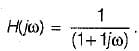When it is subjected to an input white noise process with a constant spectral dencity ‘A’ the spectral density of the output will be

Solution:
QUESTION: 6

The auto -correlation of a wide sense stationary random process is given by e-2|τ|. The peak valve of the spectral density is

Solution:
QUESTION: 7

Which one of the following is the correct fourier transform of the unit step signal?
u(t) = 1 for t > 0
= 0 for t < 0

Solution:
QUESTION: 8

The fourier transform of a conjugate symmetric function is always

Solution:
QUESTION: 9

The inverse fourier transform of sgn(f)

Solution: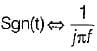then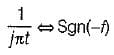∴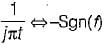∴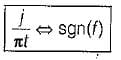QUESTION: 10

Fourier transform f(jω) of an arbitrary signal has the property

Solution:Use Code STAYHOME200 and get INR 200 additional OFF Use Coupon Code